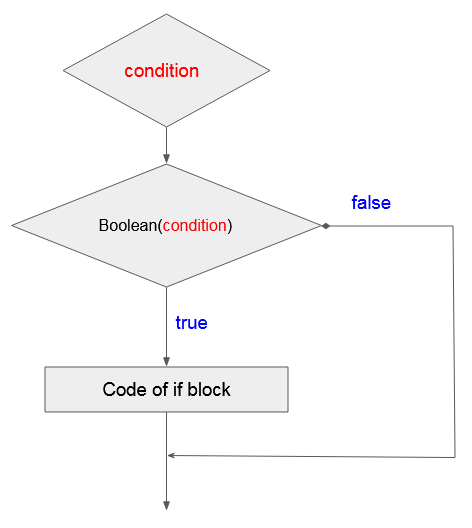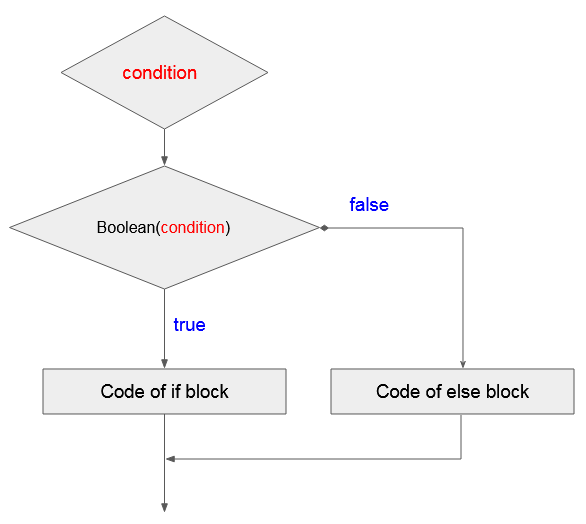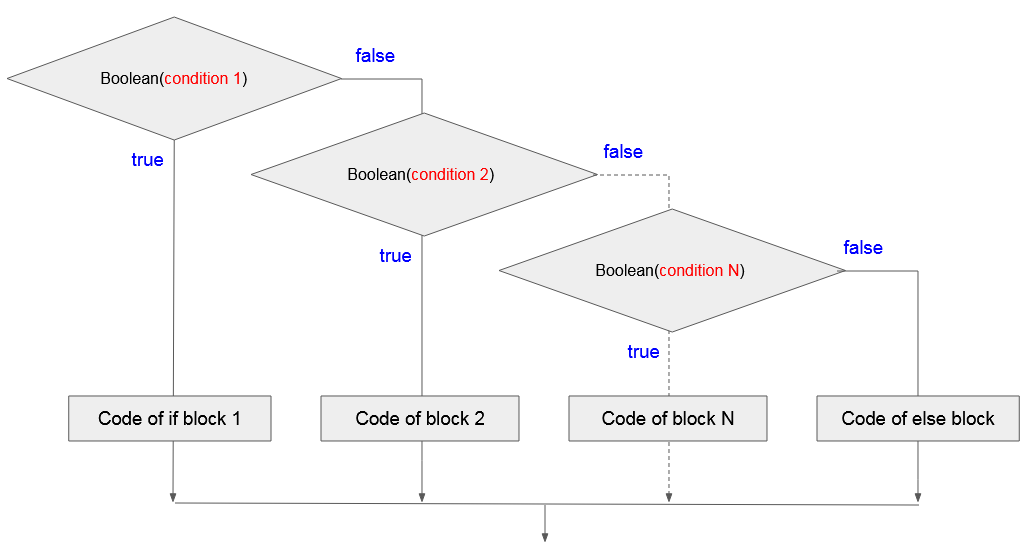# JavaScript if else Statement Tutorial with Examples

## 1. if statment

In ECMAScript, the if(condition) statement evaluates a condition to decide whether its block is executed or not.
Below is the structure of if statement:
``````if (condition)  {
// Do something here!
}``````
condition can be any value, any type or any expression. ECMAScript uses the Boolean(condition) function to evaluate. This function returns true or false.
Function: Boolean(condition)?
The Boolean(condition) function returns false if the condition has the value such as false, 0, "", null, undefined, NaN, Number.Infinite. On the contrary, this function returns true.
The following figure illustrates how to evaluate a condition of ECMAScript:Example:
simple-if-example.js
``````if(true)  { // Work
console.log("Test 1");
}

if(false) { // Not work!
console.log("Test 2");
}``````
Output:
``Test 1``
if-example.js
``````if(true)  { // Work
console.log("if(true)");
}
if(false)  { // Not work!
console.log("if(false)");
}
if('true')  { // Work
console.log("if('true')");
}
if('false')  { // Work
console.log("if('false')");
}

var obj = new Object();
if(obj)  { // Work
console.log("if(obj)");
}
if('') { // Not work!
console.log("if('')");
}
if(undefined) { // Not work!
console.log("if(undefined)");
}
if(0)  { // Not work!
console.log("if(0)");
}``````
Output:
``````if(true)
if('true')
if('false')
if(obj)``````
if-example2.js
``````var number0 = 0;
if(number0)  { // Not work!
console.log("number 0 (primitive)");
}
if(false)  { // Not work!
console.log("boolean false (primitive)");
}

// A not null Object
var myObj = {};
if(myObj)  { // Work!
console.log("A not null Object");
}
// A not null Object
var numberObject = new Number(0);
if(numberObject)  { // Work!
console.log("A not null Object (Number)");
}
// A not null Object
var booleanObject = new Boolean(false);
if(booleanObject)  { // Work!
console.log("A not null Object (Boolean)");
}``````
Output:
``````A not null Object
A not null Object (Number)
A not null Object (Boolean)``````
if-example3.js
``````// Declare a variable, representing your age
let age = 30;

// The condition to test is 'age> 20'
if (age > 20) {
console.log("Okey!");
console.log("Age is greater than 20");
}
// The code blow the 'if' block.
console.log("Done!");``````
Output:
``````Your age: 30
Okey!
Age is greater than 20
Done!``````

## 2. if - else statement

The if-else command is also used to check a condition. ECMAScript uses the Boolean(condition) function to evaluate conditions. If the evaluation result is true, the if block will be executed. On the contrary, the else block will be executed.
``````if( condition )  {
// Do something here
}
// Else
else  {
// Do something here
}``````Example:
if-else-example.js
``````// Declare a variable, representing your age
let age = 15;
// The condition to test is 'age> 18'
if (age >= 18) {
console.log("Okey!");
console.log("You are accepted!");
} else {
console.log("Sorry!");
console.log("Age is less than 18");
}
// The code after the 'if' block and 'else' block.
console.log("Done!");``````
Output:
``````Your age: 15
Sorry!
Age is less than 18
Done!``````

## 3. if - else if - else statement

The structure of an if - else if - else statement is:
``````if(condition 1)  {
// Do something here
} else if(condition 2) {
// Do something here
} else if(condition 3) {
// Do something here
}
// Else
else  {
// Do something here
}``````if-elseif-else-example.js
``````// Declaring a varible
let score = 70;

// If the score is less than 50
if (score < 50) {
console.log("You are not pass");
}
// Else if the score more than or equal to 50 and less than 80.
else if (score >= 50 && score < 80) {
console.log("You are pass");
}
// Remaining cases (that is greater than or equal to 80)
else {
console.log("You are pass, good student!");
}``````
Output:
``````Your age: 15
Sorry!
Age is less than 18
Done!``````
Change the value of the "score" variable in the above example and run the example again.
``let score = 20;``
Output:
``````Your score =20
You are not pass``````

## 4. Operators involved in conditional expression

This is a list of operators, commonly used in conditional expressions.
• > Greater Than
• < Less Than
• >= Greater Than or Equal To
• <= Less Than or Equal To
• && AND
• || OR
• == HAS A VALUE OF
• != Not Equal To
• ! NOT
Example:
if-elseif-else-example2.js
``````// Declare a variable, represents your age.
let age = 20;
// Test if age less than or equal 17
if (age <= 17) {
console.log("You are 17 or younger");
}
// Test age equals 18
else if (age == 18) {
console.log("You are 18 year old");
}
// Test if age greater than 18 and less than 40
else if (age > 18 && age < 40) {
console.log("You are between 19 and 39");
}
// Remaining cases (Greater than or equal to 40)
else {
// Nested if statements
// Test age not equals 50.
if (age != 50) {
console.log("You are not 50 year old");
}
// Negative statements
if (!(age == 50)) {
console.log("You are not 50 year old");
}
// If age is 60 or 70
if (age == 60 || age == 70) {
console.log("You are 60 or 70 year old");
}
}``````
Output:
``You are between 19 and 39``
You can change the value of the 'age' variable and run the example again to see the result.